StatLect

# Reduced row echelon form

A matrix is said to be in reduced row echelon form when it is in row echelon form and its basic columns are vectors of the standard basis (i.e., vectors having one entry equal to 1 and all the other entries equal to 0).

When the coefficient matrix of a linear system is in reduced row echelon form, it is straightforward to derive the solutions of the system from the coefficient matrix and the vector of constants.## Prerequisites

In order to understand this lecture, you should first read the lecture on the Row echelon form.

In particular, remember that a matrix is in row echelon form if and only if:

• all its non-zero rows have an entry, called pivot, that is non-zero and has only zero entries below it and to its left;

• zero-rows (if there are any) are below the non-zero rows.

When a column of a matrix in row echelon form contains a pivot, it is called a basic column. When it does not contain a pivot, we say that it is a non-basic column.

Example DefineThe matrixis in row echelon form. Its pivots are the underlined entries,,. All the columns ofare basic. There is not any non-basic column.

Example The matrixis in row echelon form. Its pivots areand. The first and third column are basic, the second is non-basic.

## Definition of reduced row echelon form

A precise definition of reduced row echelon form follows.

Definition We say that a matrix is in reduced row echelon form if and only if it is in row echelon form, all its pivots are equal to 1 and the pivots are the only non-zero entries of the basic columns.

We show some matrices in reduced row echelon form in the following examples.

Example The matrixis in reduced row echelon form. It has one zero row (the third), which is below the non-zero rows. The first and the second row are non-zero, but have a pivot (and, respectively). The two pivots are equal toand they are the only non-zero entries in their respective columns.

Example The matrixis in row echelon form because both of its rows have a pivot. However, it is not in reduced row echelon form because there is a non-zero entry in the column of the pivot.

Example The matrixis in reduced row echelon form. Its zero rows are below the non-zero rows. The first and the second row are non-zero, but have a pivot (and, respectively). The pivots are equal toand they are the only non-zero entries in their respective columns.

Example The identity matrixis in reduced row echelon form.

## How to solve a system in reduced echelon form

Consider a linear systemwhereis amatrix of coefficients,is anvector of unknowns, andis avector of constants.

The system is said to be in reduced row echelon form if the matrixis in reduced row echelon form.

As explained in the lecture on Matrix multiplication and linear combinations, the productcan be written as a linear combination of the columns of: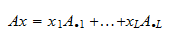where the coefficients of the combination are the unknowns.

If an unknown multiplies a basic column, it is called a basic variable. Otherwise, if it corresponds to a non-basic column, it is called a non-basic variable.

Example Define a system whose matrix of coefficientsis in reduced row echelon form, andThen,is non-basic andandare basic.

Since the reduced echelon form is a special case of the echelon form, the conditions for the existence of a solution of a system in the latter form apply, and we can use the back-substitution algorithm to solve the system.

Remember how the back-substitution algorithm works:

1. if there arebasic columns, we choosevalues arbitrarily for the non-basic variables (i.e., for the unknowns corresponding to the non-basic columns);

2. for, if the-th row is non-zero andis the basic variable corresponding to the pivot of the-th row, we setIfis in reduced row echelon form, then, so that equation 1 becomesFurthermore, the coefficientsin equation 2 are equal towhenis the index of a basic column.

Example Consider a system in reduced row echelon form with augmented matrix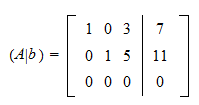Since the third column is non basic,is a non-basic variable and we can choose it arbitrarily. We chooseWe skip the third row because it is zero. On the second row, we have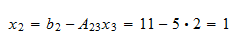and on the first one we have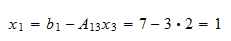## How to transform a system to reduced row echelon form

The standard algorithm used to transform a system into an equivalent system in reduced row echelon form is called Gauss Jordan elimination.

## Solved exercises

Below you can find some exercises with explained solutions.

### Exercise 1

Determine whether the matrixis in reduced row echelon form.

Solution

Let us first underline the pivots: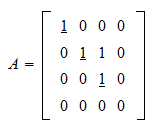Each non-zero row has a pivot. Furthermore, there is a zero row, but it is below the non-zero rows. Thereforeis in echelon form. It is not in reduced form because the third column is basic but it contains a non-zero element that is not a pivot.

### Exercise 2

Determine whether the matrix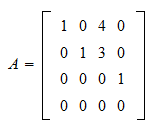is in reduced row echelon form.

Solution

Let us first underline the pivots: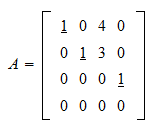Each non-zero row has a pivot. Moreover, the only zero row (the fourth) is preceded by the non-zero rows. Thereforeis in echelon form. It is also in reduced form because all the pivots are equal toand the non-pivotal elements in the basic columns are all equal to.